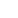We use cookies to give you the best experience on our website. If you continue to browse, then you agree to our privacy policy and cookie policy.# The problem of numerical calculation with decimal.

In the Control GridControl ,The number A1:10.251 and A2:3.575, set NumberDecimalDigits=2, the cell displays the values A1:10.25 and A2:3.58.

Then apply the formula "=A1+A2" result was 13.83. results from their raw values as 13.826.

How can I get 13.826 instead of 13.83?

7 Replies

KB Kanimozhi Bharathi Syncfusion Team November 19, 2014 07:13 PM UTC

Hi Mark,

Thank you for contacting Syncfusion Support.

We have analysed your query. If you set the Number of Decimal Digits to be 2, then it will be rounded and will be displayed with 2 decimal digits only. If you want to display 3 digits after the decimal point then set the NumberDecimalDigits to be 3.

You can also refer our Dashboard sample “Formula Range Selection Demo” which is under the below path in Syncfusion Dashboard.

Path: WPF Dashboard -> CellGrid -> Formula Support -> Formula Range Selection Demo

Please let us know if you have any queries.

Thanks

Kanimozhi B

ML Mark Li replied to Kanimozhi Bharathi November 20, 2014 02:45 AM UTC

Hi Mark,

Thank you for contacting Syncfusion Support.

We have analysed your query. If you set the Number of Decimal Digits to be 2, then it will be rounded and will be displayed with 2 decimal digits only. If you want to display 3 digits after the decimal point then set the NumberDecimalDigits to be 3.

You can also refer our Dashboard sample “Formula Range Selection Demo” which is under the below path in Syncfusion Dashboard.

Path: WPF Dashboard -> CellGrid -> Formula Support -> Formula Range Selection Demo

Please let us know if you have any queries.

Thanks

Kanimozhi B

According to what you mean,If I set the Number of Decimal Digits, It is equal to the applications of the function Round (number, num_digits)?

KB Kanimozhi Bharathi Syncfusion Team November 23, 2014 02:36 PM UTC

Hi Mark,

Apologize for the delay.

We have analyzed your query. Setting the Number of Decimal digits is not equal to the Round() function. The Round() function returns a number rounded to a specified number of digits.

For Eg: Round(23.0001,2) will return the rounded value as 23, while when we set the Number of Decimal Digits to be 2, then it returns the value as 23.00 with two decimal digits.

Please let us know if you need any clarifications.

Thanks

Kanimozhi B

ML Mark Li November 27, 2014 07:57 AM UTC

Hi Kanimozhi,Please Take a look at this picture,I open Excel input values and calculatestheir sum.A1,B1 is A2,B2 after setting up two decimal values,Can I get C1='12.728'?

Thanks
Mark Li

KB Kanimozhi Bharathi Syncfusion Team December 1, 2014 06:34 PM UTC

Hi Mark,

Apologize for the delay.

We have analyzed your query. You can get three decimal digits for the resultant value by setting the Number of Decimal digits to be 3.

For Eg:

Let us take,

A1= 10.2015 and set the NumberDecimalDigits be 2

B1 = 2.523 and  set the NumberDecimalDigits be 2

For the resultant value C1 = A1+B1, Set the NumberDecimalDigits to 3 then you can get your expected output 12.728.

Please let us know if you have any queries.

Thanks

Kanimozhi B

ML Mark Li replied to Kanimozhi Bharathi December 9, 2014 07:39 AM UTC

Hi Mark,

Apologize for the delay.

We have analyzed your query. You can get three decimal digits for the resultant value by setting the Number of Decimal digits to be 3.

For Eg:

Let us take,

A1= 10.2015 and set the NumberDecimalDigits be 2

B1 = 2.523 and  set the NumberDecimalDigits be 2

For the resultant value C1 = A1+B1, Set the NumberDecimalDigits to 3 then you can get your expected output 12.728.

Please let us know if you have any queries.

Thanks

Kanimozhi B

But,The Cell "C1" is a FormulaCell, Set the NumberDecimalDigits has no effect.If you have a example it will be even better.

Thanks
Mark Li

KB Kanimozhi Bharathi Syncfusion Team December 11, 2014 12:33 PM UTC

Hi Mark,

Apologize for the delay

We were able to reproduce the Number Format issue in Formula cell and have logged defect report regarding this. The fix for this issue will be included in any our upcoming releases.

Please let me know if you have any questions.

Thanks

Kanimozhi B